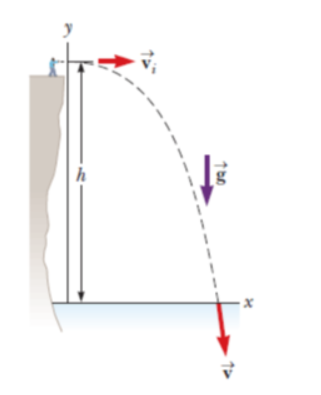# Problem: A student stands at the edge of a cliff and throws a stone horizontally over the edge with a speed of vi = 18.0 m/s. The cliff is h = 50.0 m above a body of water as shown in Figure P4.29. (a) What are the coordinates of the initial position of the stone? (b) What are the components of the initial velocity of the stone? (c) What is the appropriate analysis model for the vertical motion of the stone? particle under constant, nonzero acceleration particle under constant speed(d) What is the appropriate analysis model for the horizontal motion of the stone? particle under constant, nonzero acceleration particle under constant speed(e) Write symbolic equations for the x and y components of the velocity of the stone as a function of time. (f) Write symbolic equations for the position of the stone as a function of time. (g) How long after being released does the stone strike the water below the cliff? (h) With what speed and angle of impact does the stone land?

###### FREE Expert Solution

In this problem, we are launching a stone horizontally. Thus, it is a projectile motion with a horizontal launch.

Uniform accelerated motion (UAM) equations, a.k.a. "kinematics equations":

90% (441 ratings)###### Problem Details

A student stands at the edge of a cliff and throws a stone horizontally over the edge with a speed of vi = 18.0 m/s. The cliff is h = 50.0 m above a body of water as shown in Figure P4.29.

(a) What are the coordinates of the initial position of the stone?

(b) What are the components of the initial velocity of the stone?

(c) What is the appropriate analysis model for the vertical motion of the stone?

• particle under constant, nonzero acceleration
• particle under constant speed

(d) What is the appropriate analysis model for the horizontal motion of the stone?

• particle under constant, nonzero acceleration
• particle under constant speed

(e) Write symbolic equations for the x and y components of the velocity of the stone as a function of time.

(f) Write symbolic equations for the position of the stone as a function of time.

(g) How long after being released does the stone strike the water below the cliff?

(h) With what speed and angle of impact does the stone land?